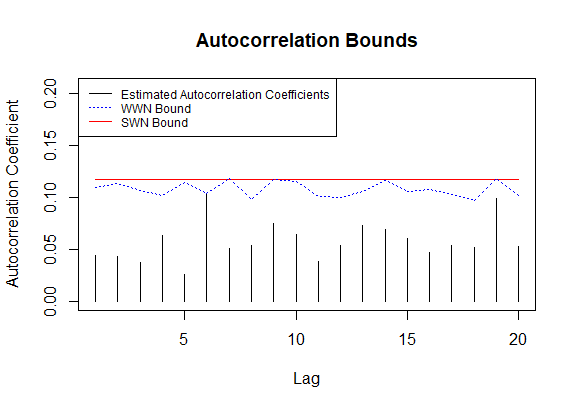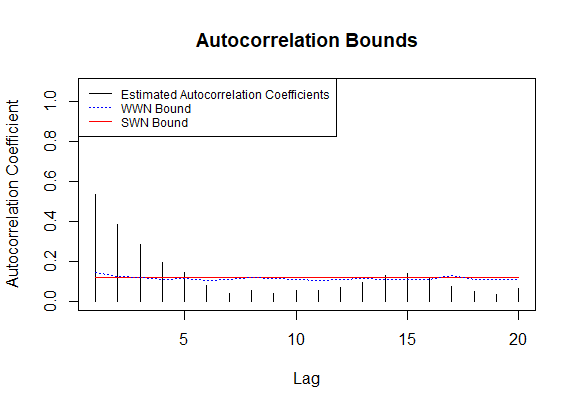# An Overview of the wwntests Package

#### 2020-05-18

This package aims to provide a variety of hypothesis tests to be used on functional data, testing assumptions of weak/strong white noise, conditional heteroscedasticity, and stationarity.

We draw up some simple expository examples with a sample of Brownian motion curves and a sample of FAR(1,0.75)-IID curves (which are conditionally heteroscedastic).

library(wwntests)
#> Registered S3 method overwritten by 'quantmod':
#>   method            from
#>   as.zoo.data.frame zoo
set.seed(1234)
b <- brown_motion(N = 200, J = 100)
f <- far_1_S(N = 200, J = 100, S = 0.75)

Note that ‘T’ denotes the number of samples (Brownian motions) and ‘J’ denotes the number of times each Brownian motion is sampled, henceforth referred to as the resolution of the data.

We denote a discretely observed functional time series of length $$T$$ by $$\{X_i(u) : 1 \le i \le T, u \in (0, 1]\} = (X_i)$$ (the parameter $$i$$ indexes the samples). Each $$X_i$$ is seen as an element of the Hilbert space of real-valued square integrable functions on the interval $$(0,1]$$.

## Single- and Multi-Lag Tests 

The autocovariance function for a given lag h is given by $$\gamma_h(t,s) = E[(X_0(t) - \mu_X(t))(X_h(s) - \mu_X(s))$$. The single-lag test tests the hypothesis $$\mathscr{H}_{0,h} : \gamma_h(t,s) = 0$$. Thus, this test is useful to identify correlation at a specified lag.

On the other hand, the multi-lag test is able to identify correlation over a range of lags. It tests the hypothesis $$\mathscr{H}_{0,K} : \forall j \in \{1, \ldots, K\} \gamma_j(t,s) = 0$$.

The tests statistics for $$\mathscr{H}_{0, h}$$ and $$\mathscr{H}_{0,K}$$ are $Q_{T, h} = T || \gamma_h ||^2 \text{ and } V_{T, K} = T \sum_{h = 1}^K ||\gamma_h||^2$ For a complete and rigorous treatment of this process, and the theory these two tests, please refer to Kokoszka, Rice, Shang .

### Applying the Single-Lag and Multi-Lag Tests to Data

We try the single-lag tests with a lag of 1, and the multi-lag test with a maximum lag of 10 (note, the default significance level is $$\alpha = 0.05$$) on our functional Brownian motion and FAR data using the fport_test function and passing the string handles ‘single-lag’ and ‘multi-lag’ to the test parameter. For the single-lag test, the lag parameter determines the lag of the of the autocovariance function, and for the multi-lag test, it determines the maximum lag to include in $$V_{T,K}$$ (that is, lag = $$K$$).

fport_test(f_data = b, test = 'single-lag', lag = 1, suppress_raw_output = TRUE)
#> Single-Lag Test
#>
#> null hypothesis: the series is uncorrelated at lag 1
#> p-value = 0.729104
#> sample size = 200
#> lag = 1
fport_test(f_data = f, test = 'single-lag', lag = 1, suppress_raw_output = TRUE)
#> Single-Lag Test
#>
#> null hypothesis: the series is uncorrelated at lag 1
#> p-value = 0.000000
#> sample size = 200
#> lag = 1
fport_test(f_data = b, test = 'multi-lag', lag = 10, suppress_raw_output = TRUE)
#> Multi-Lag Test
#>
#> null hypothesis: the series is a weak white noise
#> p-value = 0.797724
#> sample size = 200
#> maximum lag = 10
fport_test(f_data = f, test = 'multi-lag', lag = 10, suppress_raw_output = TRUE)
#> Multi-Lag Test
#>
#> null hypothesis: the series is a weak white noise
#> p-value = 0.000000
#> sample size = 200
#> maximum lag = 10

We omit any analysis of results here for the sake of brevity, however, one will see that all results are as expected given our knowledge of the underlying data generating processes.

### Visualizing the Single-Lag Test

The nature of the single-lag test allows for a simple and illustrative visualization. The autocorrelation_coeff_plot plots estimated autocorrelation coefficients, which are defined by $$\rho_h = \frac{||\gamma_h||}{\int y_0(t,t)\mu(dt)}$$, over a range of lags. It also plots confidence bounds (for a significance level $$\alpha$$) for these coefficients under weak white noise (plotted in blue) and strong white noise assumptions (constant, plotted in red). We remark that these bounds should be violated approximately $$\alpha \%$$ of the time if the underlying assumptions are satisfied. We plot the single-lag autocorrelation plots for our Brownian motion and FAR data below.

autocorrelation_coeff_plot(f_data = b, K = 20)autocorrelation_coeff_plot(f_data = f, K = 20)## The Spectral Density Test 

The single-lag test, and in particular, the multi-lag test, are computationally expensive. Another supported test, referred to by its string handle ‘spectral’, which is significantly faster. The drawback of this test, is that it is not built for general white noise (e.g. functional conditionally heteroscedastic) series. It is based on the spectral density operator $$\mathscr{F}(\omega) = \frac{1}{2\pi} \sum_{j \in \mathbb{Z}} C(j)e^{-ij\omega}, \omega \in [-\pi, \pi]$$, where $$C(j)$$ are the autocovariance operators, $$C(j) = E[X_j \otimes X_0], j \in \mathbb{Z}$$. These operators are estimated by $$\hat{C}_n(j) = \frac{1}{n} \sum_{t = j+1}^n u_t \otimes u_{t-j}, 0 \le j < n$$ and $$\hat{\mathscr{F}}_n(\omega) = \frac{1}{2\pi} \sum_{|j|<n} k(\frac{j}{p_n})\hat{C}_n(j)e^{-ij\omega}, \omega \in [-\pi, \pi]$$, where $$k$$ is a user-chosen kernel function and $$p_n$$ is the bandwidth parameter (or lag-window); it may either be a user-inputted positive integer, computed from the sample size via $$p_n = n^{\frac{1}{2q+1}}$$, or computed via a data-adaptive process (see Characiejus, Rice ). Currently supported kernel functions are the Bartlett and Parzen kernels: \begin{align*} k_B(x) &= \begin{cases} 1 - |x| & \text{ for } |x| \le 1 \\ 0 & \text{ otherwise } \end{cases} & \text{(Bartlett)} \\ k_P(x) &= \begin{cases} 1 - 6x^2 + 6|x|^3 & \text{ for } 0 \le |x| \le \frac{1}{2} \\ 2(1 - |x|)^3 & \text{ for } \frac{1}{2} \le |x| \le 1 \\ 0 & \text{ otherwise } \end{cases} & \text{(Parzen)} \end{align*}

We then consider the the distance $$Q$$ (in terms of integrated normed error) between the spectral density operator $$\mathscr{F}(\omega), \omega \in [-\pi, \pi]$$ and $$\frac{1}{2\pi}C(0)$$: $Q^2 = 2 \pi \int_{-\pi}^{\pi} || \mathscr{F}(\omega) - \frac{1}{2\pi}C(0)||_2^2 d \omega$ The test statistic is: $T_n = T_n(k, p_n) = \frac{2^{-1} n \hat{Q}_n^2 - \hat{\sigma}_n^4C_n(k)}{||\hat{C}_n(0)||_2^2 \sqrt{2D_n(k)}}, n \ge 1$ , where $$\hat{\sigma}^2_n = n^{-1} \sum_{t=1}^n ||X_t||^2$$, $$C_n(k) = \sum_{j=1}^{n-1}(1 - \frac{j}{n})k^2(\frac{j}{p_n})$$, and $$D_n(k) = \sum_{j=1}^{n-2} (1 - \frac{j}{n})(1 - \frac{j+1}{n})k^4(\frac{j}{p_n})$$. We actually use a power transformation of this test statistic proposed by Chen and Deo , but this is quite involved and we will omit this (see , ).

### Applying the Spectral Density Test to Data

We apply the spectral density test to our Brownian motion and FAR data with some different parameter configurations for illustration.

fport_test(b, test='spectral', bandwidth = 'static', suppress_raw_output = TRUE)
#> Spectral Test
#>
#> null hypothesis: the series is iid
#> p-value = 0.926583
#> sample size = 200
#> kernel function = Bartlett
#> bandwidth = 5.848035
#> bandwidth selection = static
fport_test(b, test='spectral', kernel = 'Bartlett', bandwidth = 3, suppress_raw_output = TRUE)
#> Spectral Test
#>
#> null hypothesis: the series is iid
#> p-value = 0.811402
#> sample size = 200
#> kernel function = Bartlett
#> bandwidth = 3.000000
#> bandwidth selection = 3
fport_test(f, test='spectral', kernel = 'Parzen', bandwidth = 10, suppress_raw_output = TRUE)
#> Spectral Test
#>
#> null hypothesis: the series is iid
#> p-value = 0.000000
#> sample size = 200
#> kernel function = Parzen
#> bandwidth = 10.000000
#> bandwidth selection = 10
fport_test(f, test='spectral', bandwidth = 'adaptive', alpha = 0.01, suppress_raw_output = TRUE)
#> Spectral Test
#>
#> null hypothesis: the series is iid
#> p-value = 0.000000
#> sample size = 200
#> kernel function = Bartlett
#> bandwidth = 16.840540
#> bandwidth selection = adaptive

## Independence Test 

Performs a test for independence and identical distribution of functional observations. The test relies on a dimensional reduction via a projection of the data on the K most important functional principal components. The empirical autocovariance operator is given by $C_N(x) = \frac{1}{N} \sum_{n=1}^N \langle X_n x \rangle X_n, x \in L^2[0,1)$ , (where $$N$$ is the sample size) and the (empirical) eigenelements of $$C_N$$ are defined by $C_N(v_{j,N}) = \lambda_j v_{j,N}, j \ge 1$ Note, the (non-empirical) eigenfunctions $$v_{j}$$ form an orthonormal basis of $$L^2[0,1)$$, and we assume $$\lambda_{1,N} \ge \lambda_{2,N} \ge \ldots$$, which are all non-negative. We decompose our functional data into its $$p$$ most important principal components: $X_n(t) = \sum_{k=1}^{p} X_{k,n} v_{k,N}$ , where $$X_{k,n} = \int_0^1 X_n(t) v_{k,N}(t)$$ Let $$\mathbf{C_h}$$ denote the sample autocovariance matrix with entries: $c_h(k,l) = \frac{1}{N} \sum_{t = 1}^{N-h} X_{k,t}X_{l, t+h}$ Letting $$r_{f,h}(i,j)$$ and $$r_{b,h}(i,j)$$ denote the $$(i,j)$$ entries of $$\mathbf{C_0}^{-1} \mathbf{C_h}$$ and $$\mathbf{C_h} \mathbf{C_0}^{-1}$$, respectively, we define the test statistic: $Q_n = N \sum_{h = 1}^H \sum_{i,j = 1}^p r_{f,h}(i,j) r_{b,h}(i,j)$ , which, under suitable conditions, converges to a $$\chi^2_{p^2 H}$$ distribution under the null hypothesis. See Gabrys, Kokoszka .

### Applying the Independence Test to Data

The ‘components’ parameter (denoted by p above) determines how many functional principal components to use (kept in order of importance, which is determined by the proportion of the variance that each computed component explains). The ‘lag’ parameter (denoted by H above) determines the maximum lag to consider. We apply the independence test to our Brownian motion and FAR data.

fport_test(b, test = 'independence', components = 3, lag = 3, suppress_raw_output = TRUE)
#> Independence Test
#>
#> null hypothesis: the series is iid
#> p-value = 0.820193
#> number of principal components = 3
#> maximum lag = 3
fport_test(f, test = 'independence', components = 16, lag = 10, suppress_raw_output = TRUE)
#> Independence Test
#>
#> null hypothesis: the series is iid
#> p-value = 0.000087
#> number of principal components = 16
#> maximum lag = 10

## General Remarks

### Suppressing Output

The main hypothesis function fport_test, as well as all the individual test functions may return two forms of output. In the default configuration, when suppress_raw_output and suppress_print_output are given as FALSE, each function will first print to the console the name of the test, the null hypothesis being tested, the p-value of the test, the sample size of the functional data, and additional information that may be unique to the given test. It will then return a list containing the p-value, the value of the test statistic, and the quantile of the respective limiting distribution. Passing suppress_print_output = TRUE will cause the function to omit any output to the console. Passing suppress_raw_output = TRUE will cause the function to not return the list. At least one of these parameters must be TRUE.